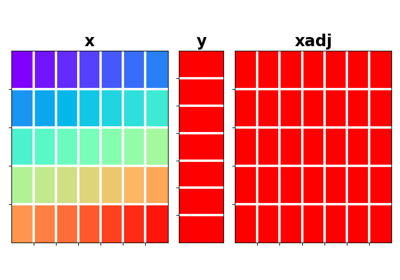# pylops.Sum#

class pylops.Sum(dims, axis=-1, forceflat=None, dtype='float64', name='S')[source]#

Sum operator.

Sum along axis of a multi-dimensional array (at least 2 dimensions are required) in forward model, and spread along the same axis in adjoint mode.

Parameters
dimstuple

Number of samples for each dimension

axisint, optional

New in version 2.0.0.

Axis along which model is summed.

forceflatbool, optional

New in version 2.2.0.

Force an array to be flattened after rmatvec. Note that this is only required when len(dims)=2, otherwise pylops will detect whether to return a 1d or nd array.

dtypestr, optional

Type of elements in input array.

namestr, optional

New in version 2.0.0.

Name of operator (to be used by pylops.utils.describe.describe)

Notes

Given a two dimensional array, the Sum operator re-arranges the input model into a multi-dimensional array of size dims and sums values along axis:

$y_j = \sum_i x_{i, j}$

$x_{i, j} = y_j \quad \forall i=0, N-1$
Attributes
shapetuple

Operator shape

explicitbool

Operator contains a matrix that can be solved explicitly (True) or not (False)

Methods

 __init__(dims[, axis, forceflat, dtype, name]) adjoint() apply_columns(cols) Apply subset of columns of operator cond([uselobpcg]) Condition number of linear operator. conj() Complex conjugate operator div(y[, niter, densesolver]) Solve the linear problem $$\mathbf{y}=\mathbf{A}\mathbf{x}$$. dot(x) Matrix-matrix or matrix-vector multiplication. eigs([neigs, symmetric, niter, uselobpcg]) Most significant eigenvalues of linear operator. matmat(X) Matrix-matrix multiplication. matvec(x) Matrix-vector multiplication. reset_count() Reset counters rmatmat(X) Matrix-matrix multiplication. rmatvec(x) Adjoint matrix-vector multiplication. todense([backend]) Return dense matrix. toimag([forw, adj]) Imag operator toreal([forw, adj]) Real operator tosparse() Return sparse matrix. trace([neval, method, backend]) Trace of linear operator. transpose()

## Examples using pylops.Sum#Sum

Sum